# How to Count Cells Are Not Blank or Empty in Excel

This post will guide you how to count cells that are not blank or empty in a given range cells using a formula in Excel 2013/2016.How do I count the number of cells that are not blank in a particular range with a VBA macro in Excel. You should know that excel offers several count functions to count the number of cells within a specific range, that meet the defined criteria or conditions in Excel.

If you want to count the number of cells that are not blank or non-empty in a selected range, you can use the COUNTA function or COUNTIF function.

## Method 1: Count Non-Blank or Non-empty Cells with COUNTA Function

Assuming that you have a data list in a range A1:A6, and this range has blank cells as well. And you wish to count cell without blank cells. And you can use a formula based on the COUNTA function like this:

=COUNTA(A1:B6)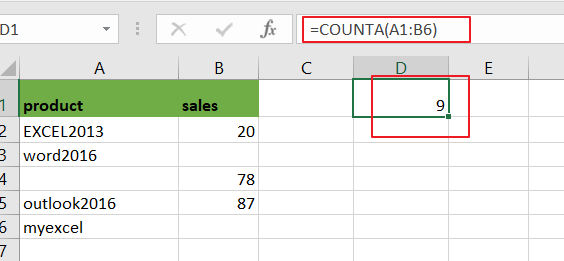The COUNTA function can be used to count the number of cells that contain numbers, text,dates or errors. And it will ignore all empty cells automatically.

## Method 2: Count Non-Blank or Non-empty Cells with COUNTIF Function

You can also use another function called COUNTIF function to achieve the same result of counting the number of cells that are not blank in Excel. For example, using the following formula:

=COUNTIF(A1:B6,”<>”)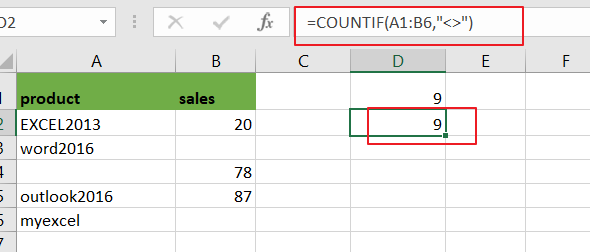From the above image, you would see that using the COUNTIF function to count the number of non-blank or non-empty cells in the range A1:B6 and return the same results as COUNTA function.

## Method 3: Count Non-Blank or Non-empty Cells with VBA Macro

You can also create an newly VBA Macro to count cells that are not empty in a given range in Excel. just do the following steps:

Step1# open your excel workbook and then click on “Visual Basic” command under DEVELOPER Tab, or just press “ALT+F11” shortcut.Step2#  then the “Visual Basic Editor” window will appear.

Step3# click “Insert” ->”Module” to create a new module.Step4# paste the below VBA code into the code window. Then clicking “Save” button.

```Sub CountCellNotBlank()
Dim ws As Worksheet
Set ws = Worksheets("Sheet7")
ws.Range("D3") = Application.WorksheetFunction.CountA(ws.Range("A1:B6"))
End Sub

```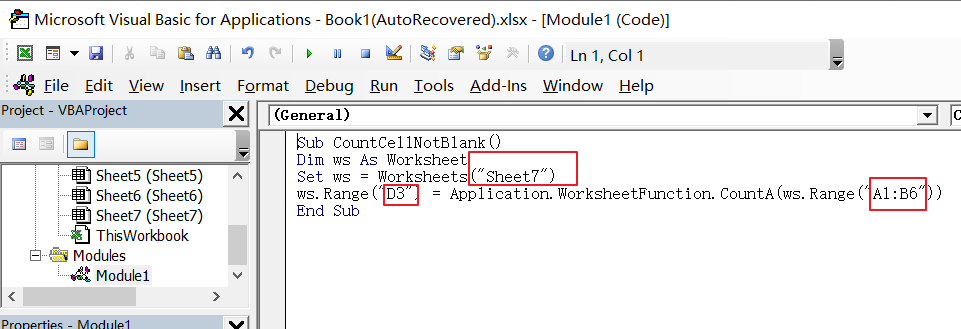Step5: back to the current worksheet, click on `Macros` button under `Code` group. then click `Run` button.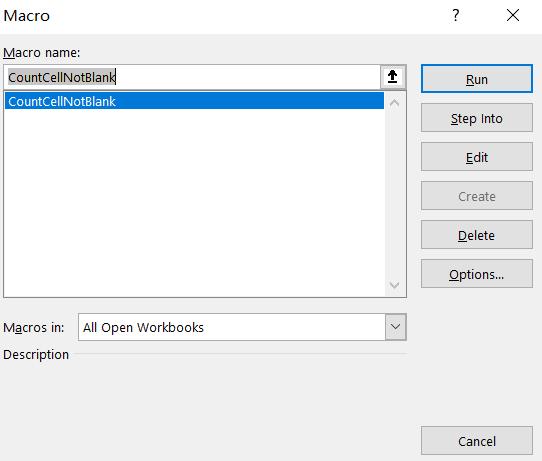Step6: let’s see the result: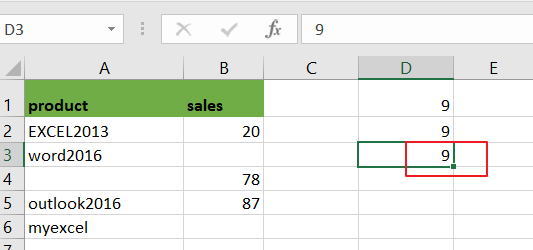### Related Functions

• Excel COUNTIF function
The Excel COUNTIF function will count the number of cells in a range that meet a given criteria. This function can be used to count the different kinds of cells with number, date, text values, blank, non-blanks, or containing specific characters.etc.= COUNTIF (range, criteria)…
• Excel COUNTA function
The Excel COUNTA function counts the number of cells that are not empty in a range. The syntax of the COUNTA function is as below:= COUNTA(value1, [value2],…)…